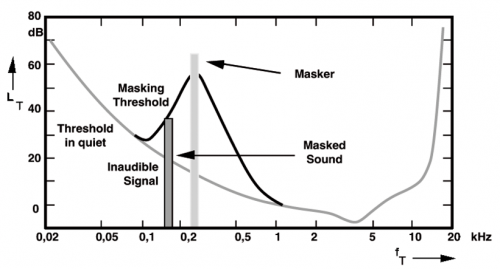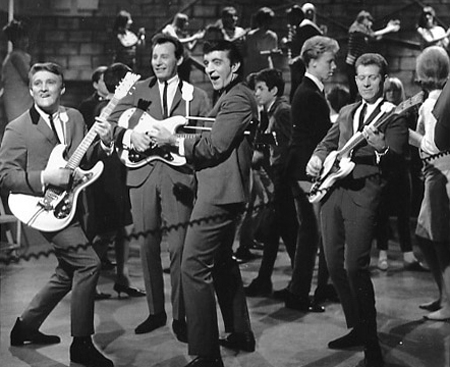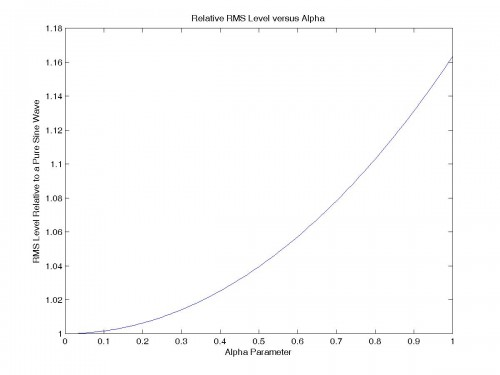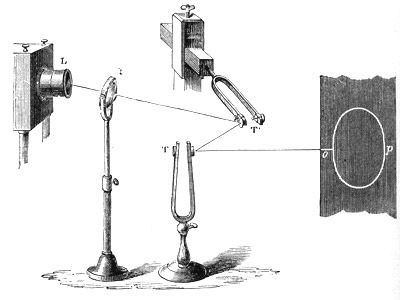## Archive for the ‘Psychoacoustics’ Category

Thursday, December 22nd, 2011In the previous post I mentioned the importance of level matching when comparing audio equipment with differing amounts of distortion.  Implicit was the assumption that the loudness of the two levels would be matched as long as the RMS levels were made equal.  Unfortunately, a psychoacoustic phenomena called auditory masking comes into play to ruin this simple picture.  Many people know that in order to double the perceived loudness of a single tone, you must increase the power by approximately ten times (+10dB).  However, to double the perceived loudness of a tone by adding a second tone at a much higher frequency, you need only double the power (+3dB)!  The reason for this is the compression from auditory masking in the cochlea.

What does this mean for level matching in the presence of distortion?  It means that to double the perceived loudness of a single tone it will take +10dB from a “distortionless” amplifier and less than +10dB from an amplifier with distortion!  This is one of the reasons that tube amplifiers are perceived as having higher power than a comparable solid-state design: The masking effect of our hearing applies less compression to the distorted sound, as the energy is spread over a broader frequency range.  Does this mean tube amps are inherently flawed, inaccurate, etc?  No, like they say “The proof of the pudding is in the eating.”  No amount of hand-waving from audio purists will ever sway some folks from their tube amps and there’s nothing wrong with that.

There are mathematical models available for this compression process, but when coupled with the typical transfer functions of real audio equipment (not just the simple single transistor example I gave in the previous post), there are no nice closed-form solutions that can be used for accurate auditory level matching.  This is something that could be done with simulation however.  It might be worthwhile trying it to see what types of distortion mechanisms give the highest level of perceived loudness versus RMS level (it seems like higher order distortion would, but this is known to give poor sound quality – I suppose it is loud though).  Overall, the best solution for now is the classic one: Live with a piece of gear for a while to get a good feel for it.

### Euphonic Distortion?

Tuesday, May 17th, 2011Why is it that every time you mention that a piece of audio equipment sounds better than another, the spectre of “euphonic distortion” is invoked?  Is it possible that it is really that simple?  You just add some 2nd harmonic distortion and poof!  Now it sounds better?

Enough hand waving already.  They say low order harmonic distortion makes it sound better, I say it doesn’t.  Who’s right?  Well everybody prepare yourselves because…That’s right, science!  And there’s nothing you can do to stop me – mwaah ha ha ha!

First off let’s start simple.  Some folks say it sounds better when you add low order harmonic distortion.  One of these words in particular stands out to me: “add”.  Why does this word in particular jump out?  It’s due to the importance of level matching.  Most audiophiles know how critical it is to accurately match audio equipment if there’s any hope to perform a fair comparison, but it seems many people have forgotten in this one area.

If you add distortion, then you must adjust the level so that the RMS level matches that of the original signal!

As a concrete example, let’s consider a very simple audio amplifier: A single bipolar junction transistor.  Here’s an outline of the process to follow:

• Determine the appropriate function of the distortion mechanism
• Determine the average value in order to remove any dc component
• Determine the RMS value and apply a multiplier to compensate

Here is the equation for the single bipolar transistor stage when passing a sine wave of angular frequency$\omega$:$f(t)=\frac{1}{\alpha }\exp(\alpha \sin(\omega t))$

Where$\alpha$ is the degree of nonlinearity – i.e. lower distortion for smaller$\alpha$ and higher distortion for larger$\alpha$.

Integrating over a full cycle to get the average (i.e. dc) value:$f_{AVG}=\frac{1}{2\pi }\int_{0}^{2\pi }\frac{1}{\alpha }\exp(\alpha \sin(x))dx=\frac{1}{\alpha}I_{0}(\alpha)$

Where$I_{0}(\alpha)$ is a modified Bessel function of the first kind.  Now subtract this dc component from the original equation for the transistor stage to get the ac value:$f_{AC}(t)=\frac{1}{\alpha }(\exp(\alpha \sin(\omega t))-I_{0}(\alpha))$

From this the RMS value can now be calculated.  Here is a general expression for calculating the RMS value of a function:$f_{RMS}=\left [ \frac{1}{t_{2}-t_{1}}\int_{t_{1}}^{t_{2}} f^{2}(t)dt \right ]^{1/2}$

Now using this to calculate the RMS value of the single stage bipolar transistor when passing a sine wave:$f_{RMS}=\left [ \frac{1}{2\pi }\int_{0}^{2\pi}f_{AC}^{2}(t)dt \right ]^{1/2}=\frac{1}{\alpha}\left [I_{0}(2\alpha)-I_{0}^{2}(\alpha) \right ]^{1/2}$

Here’s a plot of this$f_{RMS}$ function versus the distortion parameter$\alpha$:So now to make the RMS level of the distorted sine wave equal to that of the undistorted sine wave, you must multiply the amplitude of the pure sine wave by the above$f_{RMS}$ expression.  You could instead divide the distorted sine wave by the same value – the point is to make the two RMS levels equal.

For clarity here are the two expressions with differing distortion, but equal RMS level – again$\alpha$ is the distortion parameter (distortion → 0 as$\alpha$ → 0):$f_{UNDISTORTED}(t)=\frac{1}{\alpha}\left [I_{0}(2\alpha)-I_{0}^{2}(\alpha) \right ]^{1/2} \sin(\omega t)$$f_{DISTORTED}(t)=\frac{1}{\alpha }(\exp(\alpha \sin(\omega t))-I_{0}(\alpha))$

As a further point of interest, the nature of the distortion mechanism considered above is that of a nice descending spectrum of harmonics, as shown in the following FFT plot for$\alpha$=0.1:I’m still doing a bit more work with this, so it is by no means anywhere close to conclusive.  However, it is at least an important, and objective, difference that must be accounted for in order to compare “apples to apples” when evaluating the subjective effect of different distortion mechanisms.

### Break In Period

Friday, April 3rd, 2009Most audiophiles agree that there is a certain “break in period” required for audio gear to start sounding right.  Most non-audiophiles will agree that this is the case for something like a loudspeaker, where there is a measureable change in the driver parameters after loosening up a bit, but tend to regard break in phenomena in audio electronics with great skepticism.  Most audiophiles point to the usual suspects: resistors, capacitors, and magnetics.  This is a good start, but there is a bit more to it.

There is a great exhibit at the Exploratorium in San Francisco.  It consists of a basketball, a hoop, and a pair of funky glasses that skew your vision to one side.  You start by making a few shots without the glasses – no big deal, the hoop is fairly close.  Next you put on the glasses and every shot now goes off to one side – a very strange sensation!  However, after several shots you notice that each shot gets a little better as your brain starts to slowly adapt to this new “reality”.

Much like the illusion given by the funky glasses in the basketball exhibit, every piece of audio equipment is nothing more than part of an “illusion engine”.  Since the musical reproduction we are creating is an illusion, our brain must learn how to perceive it correctly for the illusion to work at all.  This is a significant part of the break in period for audio gear – we are breaking in, right along with the equipment!

Every piece of audio gear has errors, regardless of typical “perfect sound forever” claims.  Some errors are easier for your brain to adapt to than others.  A longer break in period may in part be the result of errors that require a bit more “training”.  Note that the amount of training needed is also a function of the individual.  Some listeners may readily forgive certain types of error (e.g. dynamic compression), while being hypersensitive to other types of error (e.g. poor imaging).

### ABX Testing and the Heisenberg Uncertainty Principle

Sunday, March 29th, 2009

ABX testing is a form of audio testing where two components (A and B) are carefully matched with respect to level, some means is included to at will switch between the two during the course of a musical passage, and the listener is completely unaware of which is which (X).  This is the “de facto” standard for serious audio testing.  It is an excellent approach in principle, however there is a serious flaw: it only allows for the detection of gross differences due to the relatively brief samples involved.

The Heisenberg Uncertainty Principle in its most common form states that:$\Delta x\Delta p\geq \frac{\hbar}{2}$

Where$\Delta x$ is the uncertainty in position,$\Delta p$ is the uncertainty in momentum, and$\hbar$ is Plank’s constant divided by$2\pi$.

There is another form that gives the same relationship for energy and time:$\Delta E\Delta t\geq \frac{\hbar}{2}$

Where$\Delta E$ is the uncertainty in energy and$\Delta t$ is the uncertainty in time.

What does this have to do with ABX testing you may ask?  Well, nothing actually, as the principle does not apply to the macroscopic world due to the extremely small value of Plank’s constant.  However, it provides insight to the issue of ABX testing.  I propose that there is a similar relationship between the perceived difference and the listening interval.  Let’s denote this as follows:$\Delta \varepsilon \Delta \tau \geq k$

Where$\Delta \varepsilon$ is the uncertainty of the listener as to whether a difference exists or not,$\Delta \tau$ is the interval during which the listener compares the two components, and$k$ is is a listener-dependent constant (i.e. it is larger for “tin ears” and smaller for “golden ears”).

The bottom line is that a given listener will be able to detect finer and finer differences between two components over time.  This means you really have to “live” with a component for some time to appreciate the subtle differences between it and another component.  Unfortunately, it is very difficult to be objective with a long term test such as this, but I have no doubt that somebody will ultimately figure out a way of doing it.

### Precedence Effect

Monday, December 15th, 2008The precedence effect is a particularly important psychoacoustic effect for audio systems.  Based on arrival time, a given sound is broken up into three distinct bands:

t < 5mS

This is the first arrival interval.  It is essential for localization.

5 mS < t < 30mS

This is the integration interval.  Any additional sound that has the same “nature” as the original will be integrated and will not affect localization information.

30mS < t

This is the interval beyond the domain of the precedence effect.  Any additional sound that has the same “nature” as the original will be perceived as a quick echo.

What does this mean for audio system design?  For pro audio applications, it allows for sound reinforcement – for example public address.  The source itself may be much lower level than the reinforcement, but as long as the source precedes the reinforcement by about 5mS to 30mS, the source will still be perceived as the origination of the sound.  For audiophile applications, it means that a loudspeaker should have minimal stored energy if there is to be any hope of presenting a stereo image.  Also, diffraction should be minimized, as this produces multiples sources with an arrival time that falls within the 5mS window – thereby confusing localization.

### Auditory Illusions

Tuesday, December 2nd, 2008Much as optical illusions teach us a tremendous amount about how our vision works, auditory illusions provide the same sort of insight as to how our hearing works.

Illusions are a great learning tool because they are both fun and memorable.  Dianna Deutsch has developed some particularly interesting and revealing auditory illusions.

The knowledge of this and other elements of psychoacoustics is essential for audio design, so put on your headphones and enjoy!